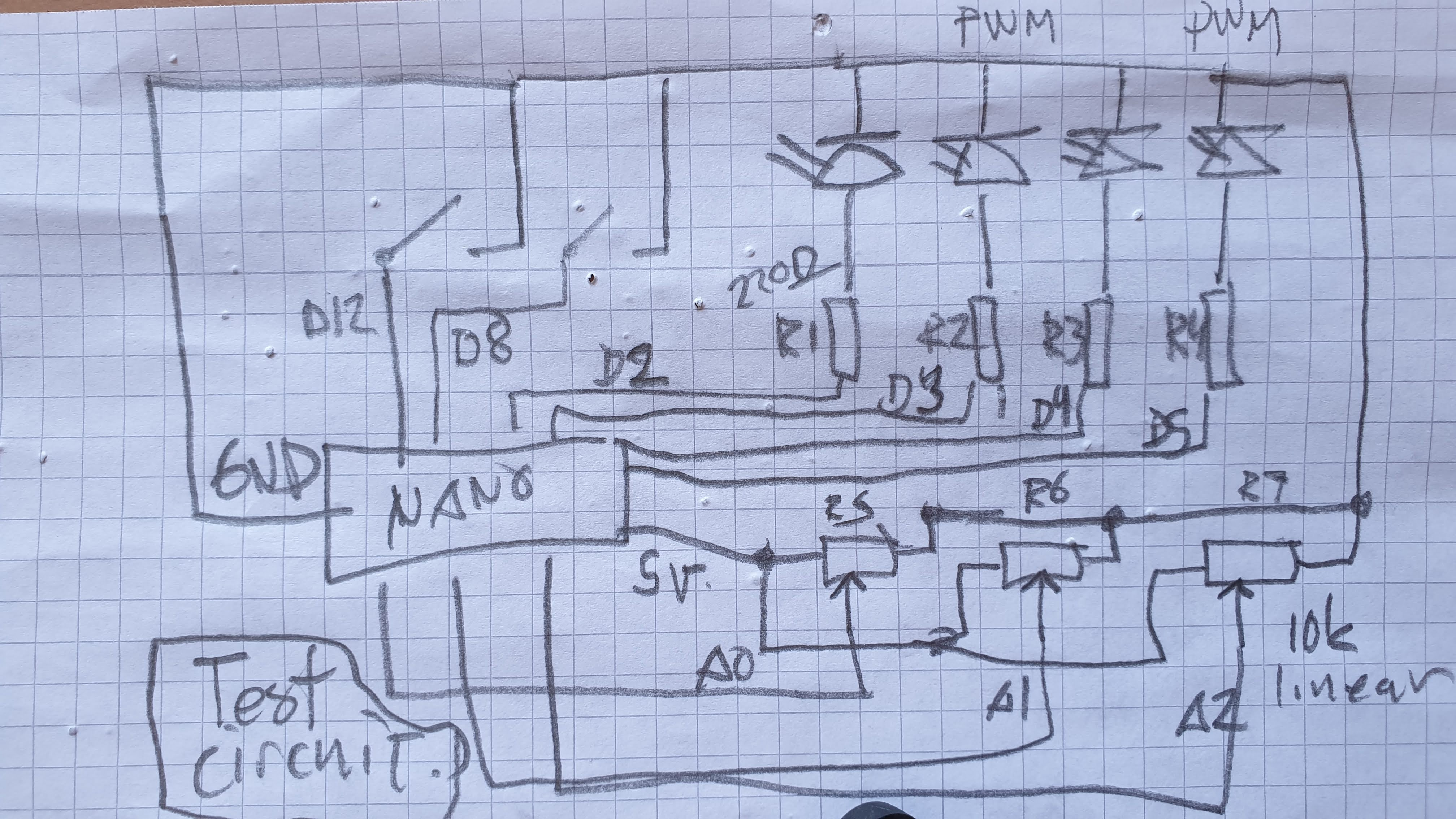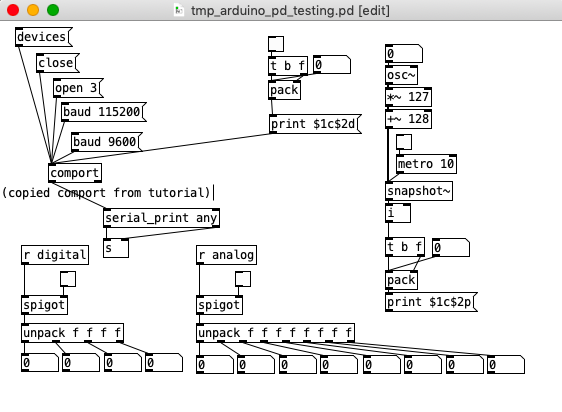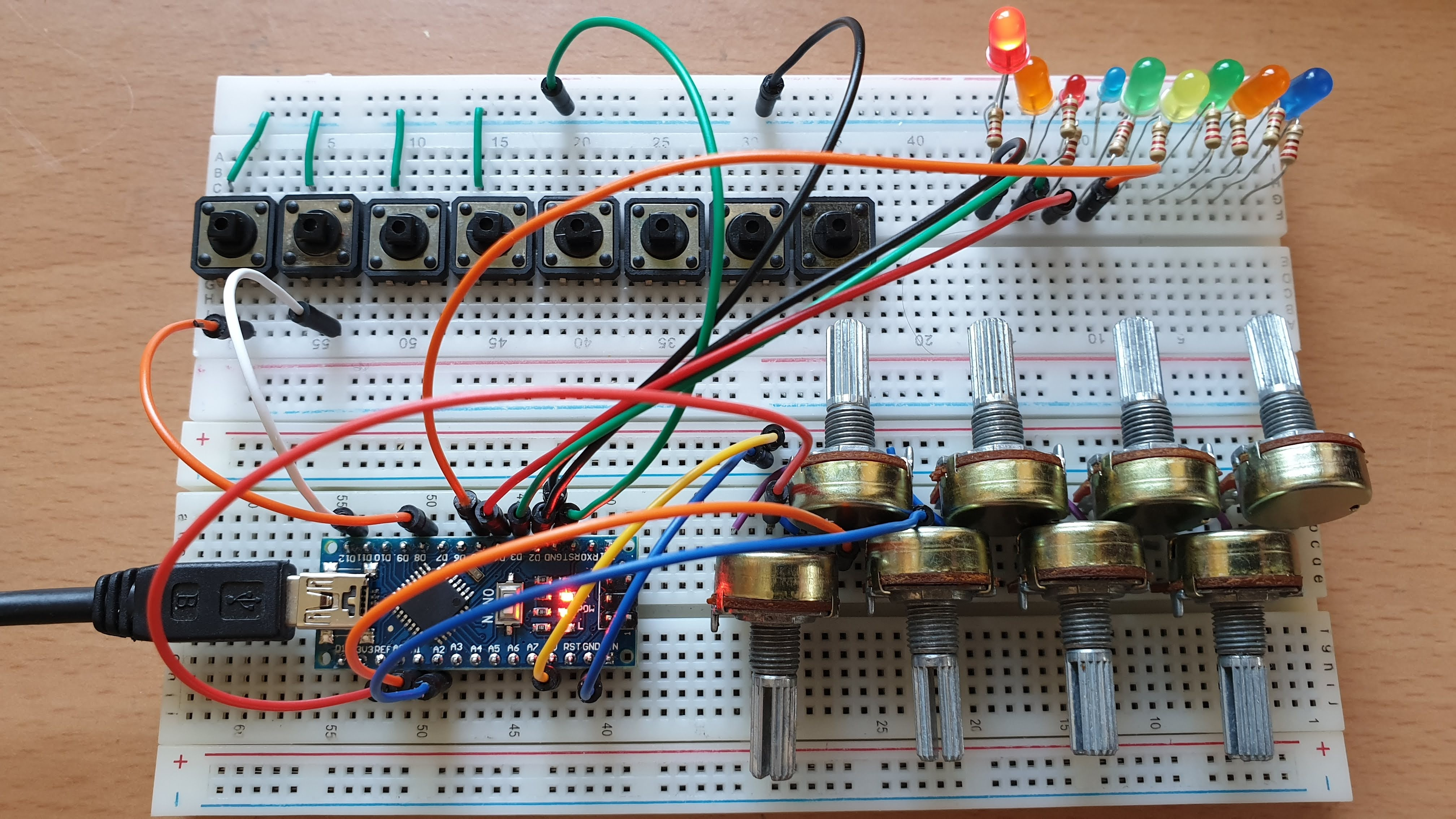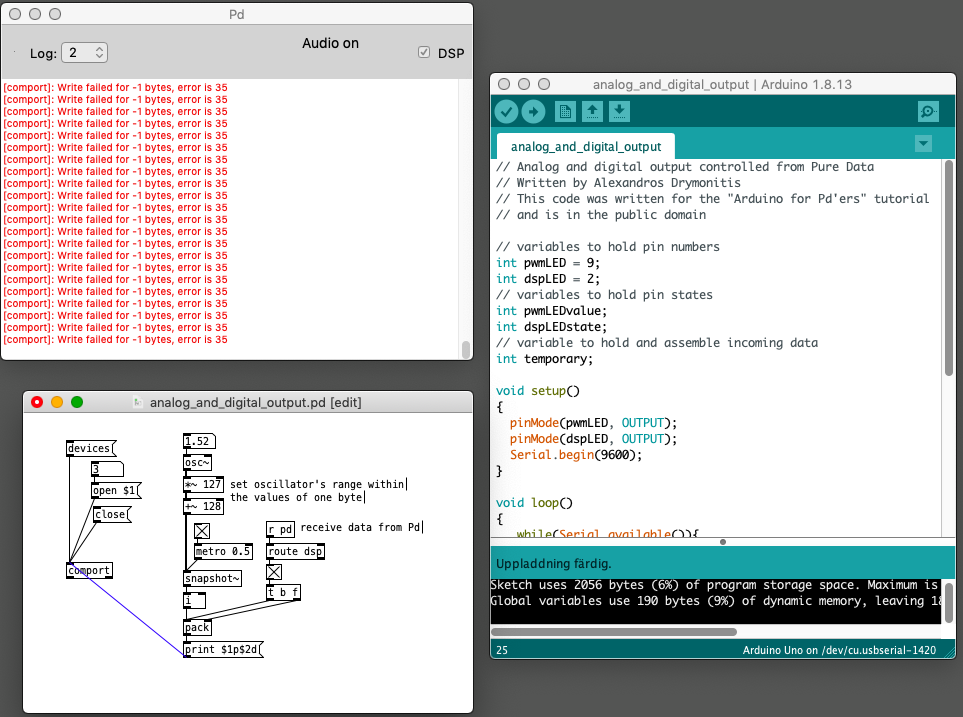• ### Having lots of switches into Pd

I have using four arduino uno in parallel with the Pduino library, using most of the digital and analog pins. As inputs. I have experienced many glitches with this setup and I have been OK with that since I am exploring and experimenting. But now I need to clean it up a bit.

What alternatives may there be instead of using arduino? Are there better solutions?

When using multiple arduinos, how to avoid problems?

Matrix switches... is this a good idea? To keep the arduino count low.

Thanks

• Posts 40 | Views 1849
• @cfry why do you call pinMode on pins A0 through A7? You don't need to declare the analog pins. Is this for potentiometers? Is there a reason you need the input_pullup resistors on these pins?
What about the input dogotal pins?Are you using resistors in your circuit? Cause you're not using the input_pullups there.
Can you share a schematic of your circuit?

• I am at the moment experimenting with different setups and circuits using heat sensitive sensors and haptic feedback (and audio).

But what I am after is to be able to choose freely whatever combination of pin and pin configuration to be used with pd, when experimenting. So there is no specific circuit. I have several different scenarios that I want to try.

The actual pin settings and modes are not important, I just hope to be able to handle digital i/o and analog i/o at the same time.

I dont know how to debug in this setup either.

Thanks for helping@alexandros said:

@cfry why do you call pinMode on pins A0 through A7? You don't need to declare the analog pins. Is this for potentiometers? Is there a reason you need the input_pullup resistors on these pins?
What about the input dogotal pins?Are you using resistors in your circuit? Cause you're not using the input_pullups there.
Can you share a schematic of your circuit?

• @alexandros

...or am I trying to use the arduino with pd in a not suitable way? Would it be better to skip on the idea of using ins and outs at the same time and instead use two (nanos), one for ins and the other for outs?

• @cfry definitely not necessary to use two Arduinos. I can't really understand where your problem lies. You didn't reply to my earlier question about the pinMode calls where you use INPUT_PULLUP on analog inputs. Is this really necessary? Also, why not use the analog pins in an array like with the digital pins? It will save a lot of writing and it's less error prone.

One thing you might want to consider is having the Arduino notify Pd when it's done sending all its data and that it's ready to receive. That could be done at the end of the loop() function like this:

``````Serial.print("done ");
Serial.println("bang");
``````

And in Pd have a [r done] object which will output a bang. Use this bang to send data from Pd to the Arduino by unpacking a list with all the accumulated data, possibly with a [list-abs/list-drip].
I use this notification technique in my 3dPdModular system, but there's a LOT of data there and it was only necessary. Perhaps you could try it out.

• Thank you for replying and sorry if I am unclear. I will try to slow down a bit.

@alexandros said:

@cfry definitely not necessary to use two Arduinos. I can't really understand where your problem lies.

-The problem is that it does not work when I try to use an arbitrary combination of pins in different modes (Digital in, Digital out, Analog in, Analog out).

Another problem is that I have a hard time reading the code parts that are handling the conversion data in order to do the serial communication, although I understand the principle of it.

You didn't reply to my earlier question about the pinMode calls where you use INPUT_PULLUP on analog inputs. Is this really necessary?

-I assumed that the problem lied in void ()loop as a conflict between the output and input handling sections. And that pinMode calls in void setup() was not relevant in this case. That is why I did not answer that question directly.

Also, why not use the analog pins in an array like with the digital pins? It will save a lot of writing and it's less error prone.

-I was foreseeing that I may want to configure those pins individually, but I can set it up with a loop for now.

One thing you might want to consider is having the Arduino notify Pd when it's done sending all its data and that it's ready to receive. That could be done at the end of the loop() function like this:

``````Serial.print("done ");
Serial.println("bang");
``````

And in Pd have a [r done] object which will output a bang. Use this bang to send data from Pd to the Arduino by unpacking a list with all the accumulated data, possibly with a [list-abs/list-drip].
I use this notification technique in my 3dPdModular system, but there's a LOT of data there and it was only necessary. Perhaps you could try it out.

-I will try that.

Since I consider this to be basically your code I assumed that you could easily just insert the code for both input and output in void loop() in a way that works and that would show me how to solve it. Then I would be able to do further explorations and it would be end of story.

I will digest this a bit and may start a new thread, since this one has slided from "Having lots of switches into Pd" to "having a working code template for communicating between Arduino and Pd using and an arbitrary mixture of pin modes".

Please let me know if this still does not make sense. Thank you so much!

• @cfry the thing is that the last bit of code you posted seems just fine, excluding the calling to pinMode where you're enabling the integrated pull-up resistors. Can you try that code removing these lines in setup(), and instead of heat sensitive sensors or whatever you're currently using, use simple potentiometers and verify whether that works or not? In case it doesn't work, please post a diagram of your circuit in some way (even hand-drawn is ok), as well as the full Arduino sketch and a screenshot of the Pd patch.

• @alexandros said:

@cfry the thing is that the last bit of code you posted seems just fine, excluding the calling to pinMode where you're enabling the integrated pull-up resistors. Can you try that code removing these lines in setup(), and instead of heat sensitive sensors or whatever you're currently using, use simple potentiometers and verify whether that works or not? In case it doesn't work, please post a diagram of your circuit in some way (even hand-drawn is ok), as well as the full Arduino sketch and a screenshot of the Pd patch.``````int outPins = {2, 4, 7};
int pwmPins = {3, 5, 6, 9, 10, 11};

int channel = 0;

int inPins = {8, 12};
int analogPins = {0, 1, 2, 3, 4, 5, 6, 7};

// int debug_skip = 0;

void setup() {

for (int i = 0; i < 3; i++) {
pinMode(outPins[i], OUTPUT);
}
for (int i = 0; i < 6; i++) {
pinMode(pwmPins[i], OUTPUT);
}
for(int i = 0; i < 2; i++) {
pinMode(inPins[i], INPUT_PULLUP);
}

Serial.begin(115200);

}

void loop() {

//DO OUTPUTS

if (Serial.available()) {

static int temp;
byte in = Serial.read();

if (isDigit(in)) temp = temp * 10 + in - '0';

//quote out this section to avoid crash

else if (in == 'c') {
channel = temp;
temp = 0;
}

else if (in == 'd') {
digitalWrite(outPins[channel], temp);
temp = 0;
}

else if (in == 'p') {
analogWrite(pwmPins[channel], temp);
temp = 0;
}

//end quote out here

}

// DO INPUTS

Serial.print("analog");

for(int i = 0; i < 8; i++){

unsigned int analogVal = analogRead (analogPins[i]);

Serial.print(" ");
Serial.print(analogVal);
}

Serial.println();

Serial.print("digital");

for(int i = 0; i < 2; i++) {

unsigned int digitalVal = digitalRead(inPins[i]);

Serial.print(" ");
Serial.print(digitalVal);
}

Serial.println();

}
``````

If I do the following PureData will always freeze and requires force quit. Occasionally the whole OS becomes sluggish/freeze and need a reboot.

1. Have this code snippet in the Arduino sketch
``````    //quote out this section to avoid crash

else if (in == 'c') {
channel = temp;
temp = 0;
}

else if (in == 'd') {
digitalWrite(outPins[channel], temp);
temp = 0;
}

else if (in == 'p') {
analogWrite(pwmPins[channel], temp);
temp = 0;
}

//end quote out here
``````
1. Connect [print \$1c\$2p] -> [comport]

(I have also noted different behavior using [comport] from the tutorial by copy and paste compared to creating a comport object (cmd+1 and then typing). At times the latter did not work at all, but after reboot of the system both seems to work.)

Thanks for helping

Here is the patch from screenshot above:
tmp_arduino_pd_testing.pd

• Have you detected whether the crash happens with `else if (in == 'd')` or `else if (in == 'p')`?
In the Pd patch it's probably better to swap the \$1 and \$2 values in the messages. Have the channel set in a cold inlet and the value in a hot one and then write this message `print \$2c\$1v`
I see in the screenshot of the patch that the PWM message is not connected to [comport]. Is this for some particular reason? Maybe the 10ms interval for sending a PWM value to [comport] is a bit too fast. Did you try something slower, like 50ms?
Another thing would be to try and merge messages for digital and PWM. Since PWM happens automatically, you could append the digital pin message and send something like `print \$2c\$1p\$4c\$3d` where \$4 will be the digital pin channel and \$3 the value.

• @alexandros said:

Have you detected whether the crash happens with `else if (in == 'd')` or `else if (in == 'p')`?

It happens only in `else if (in == 'p')`. When I bypass that I can update snapshot~ by 1 ms (I assume that 'c' will still be sent by 1 ms intervals).

In the Pd patch it's probably better to swap the \$1 and \$2 values in the messages. Have the channel set in a cold inlet and the value in a hot one and then write this message `print \$2c\$1v`

Ok I did that.

I see in the screenshot of the patch that the PWM message is not connected to [comport]. Is this for some particular reason?

It is to make sure Pd does not crash/freeze until I want to try the code.

Maybe the 10ms interval for sending a PWM value to [comport] is a bit too fast. Did you try something slower, like 50ms?

That made it work. If I use six pwm pins the limit is 150 ms before crash.

Question 1: Could there something bad that happens around `else if (in == 'p')`? In another code snippet you used this format:

``````  while(Serial.available()){
byte inByte = Serial.read();
if((inByte >= '0') && (inByte <= '9'))
temporary = 10 * temporary + inByte - '0';
else{
if(inByte == 'p'){
pwmLEDvalue = temporary;
temporary = 0;
}
else if(inByte == 'd'){
dspLEDstate = temporary;
temporary = 0;
}
}
analogWrite(pwmLED, pwmLEDvalue);
digitalWrite(dspLED, dspLEDstate);
}
``````

compared to this in the current sketch:

``````if (Serial.available()) {

static int temp;
byte in = Serial.read();

if (isDigit(in)) temp = temp * 10 + in - '0';

else if (in == 'c') {
channel = temp;
temp = 0;
}

else if (in == 'd') {
digitalWrite(outPins[channel], temp);
temp = 0;
}

else if (in == 'p') {
analogWrite(pwmPins[channel], temp);
temp = 0;
}

}
``````

Question 2: Is this crash of Pd and the computer (macOS Mojave) expected behavior in a situation like this? If it is not, maybe it should be reported as a bug. It happens consistently.

Thoughts: Could there be a problem in [comport]? This crash behavior is identical with the problems I had when using Pdunio/Firmata.

• First of all, glad it finally worked!
Answer 1: The current code looks better than the one you posted above it (which is apparently something I've used, hopefully in my tutorial and not the book...), because analogWrite() and digitalWrite() are only called when needed. In the first code snippet both functions are called whenever there's data in the serial line, which is overhead.
Answer 2: I don't really have an answer to this. You can send an email to Pd's mailing list where Martin Peach, the developer of [comport] is active. It does sound strange that your computer crashes, as a longer delay just gives time to the Arduino to do the PWM stuff, but that has to do with the Arduino. Maybe there's something strange going on when the Arduino doesn't have enough time to do what it has to do, but I really don't know.

• @alexandros said:

Answer 1: The current code looks better than the one you posted above it (which is apparently something I've used, hopefully in my tutorial and not the book...),

It is from the tutorial and I believe it was fine in a stepwise educational context. (Why I posted it was because the variables inside the else if() are treated differently. Wild guess that it could be a problem.)

Thank you so much for that you took time to help me on this. However, this problem does not exist in the old tutorial code(!).

Look hereI send two updates per millisecond and still no crash. The Pd console reports errors. Pd still works as normal. This is the codes (Arduino and Pd) from your tutorial.

When using the new codes (both Ardunio sketch and Pd patch) Pd crash/freeze and there is no report in the pd console.

Posts 40 | Views 1849
Internal error.

Oops! Looks like something went wrong!